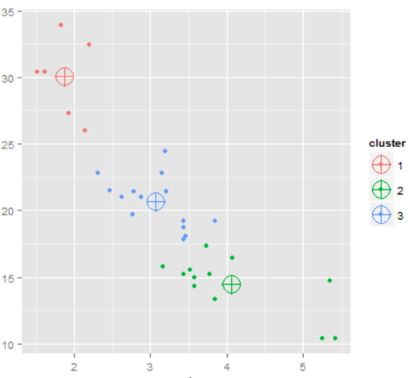[This article was first published on R - Data Science Heroes Blog, and kindly contributed to R-bloggers]. (You can report issue about the content on this page here)
Want to share your content on R-bloggers? click here if you have a blog, or here if you don't.

### Introduction

In clustering you let data to be grouped according to their similarity. A cluster model is a group of segments -clusters- containing cases (such as clients, patients, cars, etc.).

Once a cluster model is developed, one question arises: How can I describe my model?

Here we present a way to approach this question, through the implementation of Coordinate Plot in R (code available at the end of the post)

### Cluster characteristics

In general a cluster model follows:

• High similarity between cases inside the cluster.
• Each cluster should be as unique as it can, comparing with the others.

We will answer this question with one example. Each case in this data represents a country. We built a cluster model (k-means) with 3 clusters.Cluster model illustration, made of 2 variables and 3 clusters. Circles indicates the center of the cluster.

### Coordinate plot

This is the graph to describe main characteristics of cluster model:#### Coordinate plot characteristics

• Each color line represents a cluster, plus one extra line represents “All” cases.
• Each cluster has an average per each variable. And they go from 0 to 1 to be able to display all variables in one plot.
• For each variable, there will be always a number corresponding to 0 and another to 1. Because they represent the min and max value.
• Plot should be read vertical.

### How is scaled average built?

Looking at “LandArea” variable (which represents squared kilometers), we could say that C2 (cluster 2) has the lowest average regarding land area. Following by C1. C_3 has the highest value very far from the others clusters.

In other words, largest countries are in C3, while the smallest ones are in C2.

Next, there are the original values -which are not displayed- and their scaled average value:

• 1886206 is converted into: 0.17
• 243509 is converted into: 0.00
• 10014500 is converted into: 1.00

The average for the whole data (regardless clustering segmentation), is 884633 and is converted into: 0.06. That is the “All” line.

Now we’ve got our 4 points, for variable land area.

### Extracting conclusions

Describing Cluster 3

In C_3 there are the countries with the highest LandArea and Population (which are not always correlated). Regarding Energy and LifeExpectancy, they are the highest ones as well, this could be a metric of a developed country.

However they have the lowest BirthRate, it is not new that some developed countries has a low BirthRate.

Describing Cluster 2

C_2 is very similar to “All”, so there is not much information here, this cluster has averages very similar to general population.

Describing Cluster 1

C1 can be seen as the middle point regarding: LandArea, Population, Energy and Rural.
But is interesting to note that they have the highest BirthRate and the lowest LifeExpectancy, plus a high Rural variable (percentage of population living in a rural zone).
This is the opposite as C
3.

Looking at these metrics, we can write the headlines:

• C_3 => High developed countries
• C_1 => Low developed countries

### Contact

Made by Pablo C. from Data Science Heroes• This material is adapted from the e-learning course Data Science with R in which you can find step by step guide to build, understand and assess models. Request free demo at [email protected] .

• R code: Coordinate plot installation & usage available in GitHub

• Any questions regarding Data Science? Post it in our Linkedin group